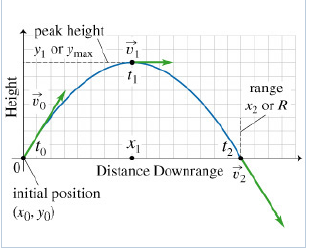# Problem: The figure (Intro 1 figure) shows the trajectory (i.e., the path) of a ball undergoing projectile motion over level ground. The time t0 = 0 s corresponds to the moment just after the ball is launched from position x0 = 0 m and y0 = 0 m. Its launch velocity, also called the initial velocity, is vvec_0. The initial velocity v0 = 30 m/s launched at an angle 60° above the positive x-axis.Two other points along the trajectory are indicated in the figure.One is the moment the ball reaches the peak of its trajectory, at time t1 with velocity v1_vec. Its position at this moment is denoted by (x1, y1) or (x1, ymax) since it is at its maximum height.The other point, at time t2 with velocity v2_vec, corresponds to the moment just before the ball strikes the ground on the way back down. At this time its position is (x2, y2), also known as (xmax, y2) since it is at its maximum horizontal range.Projectile motion is symmetric about the peak, provided the object lands at the same vertical height from which is was launched, as is the case here. Hence y2 = y0 = 0 m.What are the values of the intial velocity vector components v0,x and v0,y (both in m/s as well as the acceleration vector components a0,x and a0,y (both in m/s2? Here the subscript 0 means "at time t0." The peak of the trajectory occurs at time t1. This is the point where the ball reaches its maximum height ymax. At the peak the ball switches from moving up to moving down, even as it continues to travel horizontally at a constant rate.What are the values of the velocity vector components v1,x and v1,y (both in m/s as well as the acceleration vector components a1,x and a1,y (both in m/s2? Here the subscript 1 means that these are all at time t1.If a second ball were dropped from rest from height y_max, how long would it take to reach the ground? Ignore air resistance. Check all that apply.a) t0b) t1c) t2d) t2 - t1e) t2/2Which of the following changes would increase the range of the ball shown in the original figure? Check all that apply.a) Increase v0 above 30 m/s.b) Reduce v0 below 30 m/s.c) Reduce theta from 60° to 45°.d) Reduce theta from 60° to less than 30°.e) Increase theta from 60° up toward 90°.

###### FREE Expert Solution

Horizontal acceration, ax = 0 at all times. Therefore, the horizontal velocity, vx remains constant. Verticle acceleration, ay = -g = -9.81 m/s2 at all times. Verticle velocity decreases as the object moves upwards to zero at ymax and increases as the object moves downwards.

Velocity is a vector component.

85% (342 ratings)###### Problem DetailsThe figure (Intro 1 figure) shows the trajectory (i.e., the path) of a ball undergoing projectile motion over level ground. The time t0 = 0 s corresponds to the moment just after the ball is launched from position x0 = 0 m and y0 = 0 m. Its launch velocity, also called the initial velocity, is vvec_0. The initial velocity v0 = 30 m/s launched at an angle 60° above the positive x-axis.

Two other points along the trajectory are indicated in the figure.

• One is the moment the ball reaches the peak of its trajectory, at time t1 with velocity v1_vec. Its position at this moment is denoted by (x1, y1) or (x1, ymax) since it is at its maximum height.
• The other point, at time t2 with velocity v2_vec, corresponds to the moment just before the ball strikes the ground on the way back down. At this time its position is (x2, y2), also known as (xmax, y2) since it is at its maximum horizontal range.

Projectile motion is symmetric about the peak, provided the object lands at the same vertical height from which is was launched, as is the case here. Hence y2 = y0 = 0 m.

What are the values of the intial velocity vector components v0,x and v0,y (both in m/s as well as the acceleration vector components a0,x and a0,y (both in m/s2? Here the subscript 0 means "at time t0." The peak of the trajectory occurs at time t1. This is the point where the ball reaches its maximum height ymax. At the peak the ball switches from moving up to moving down, even as it continues to travel horizontally at a constant rate.

What are the values of the velocity vector components v1,x and v1,y (both in m/s as well as the acceleration vector components a1,x and a1,y (both in m/s2? Here the subscript 1 means that these are all at time t1.

If a second ball were dropped from rest from height y_max, how long would it take to reach the ground? Ignore air resistance. Check all that apply.

a) t0

b) t1

c) t2

d) t2 - t1

e) t2/2

Which of the following changes would increase the range of the ball shown in the original figure? Check all that apply.

a) Increase v0 above 30 m/s.

b) Reduce v0 below 30 m/s.

c) Reduce theta from 60° to 45°.

d) Reduce theta from 60° to less than 30°.

e) Increase theta from 60° up toward 90°.